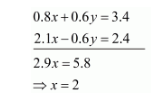# Solve the following systems of equations:

Question:

Solve the following systems of equations:

$0.4 x+0.3 y=1.7$

$0.7 x-0.2 y=0.8$

Solution:

The given equations are:

$0.4 x+0.3 y=1.7 \ldots(i)$

$0.7 x-0.2 y=0.8 \ldots(i i)$

Multiply equation (i) by 2 and equation (ii) by 3 , and add both equations we getPut the value of $x$ in equation $(i)$ we get

$0.4 \times 2+0.3 y=1.7$

$\Rightarrow 0.3 y=0.9$

$\Rightarrow y=3$

Hence the value of $x=2$ and $y=3$